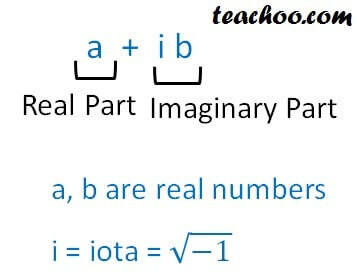Two complex numbers equal

Chapter 4 Class 11 Complex Numbers
Concept wise

Complex Numbers are numbers of the form

a + ibWhere a, b are real numbers

and i = iota = √(-1)

Learn in your speed, with individual attention - Teachoo Maths 1-on-1 Class

### Transcript

Complex Numbers are numbers of the form a + ib Real part imaginary a,b are real numbers i=iota=root-1 Where a, b are real numbers and i = iota = √(-1)